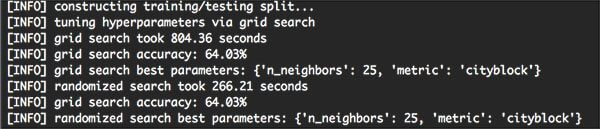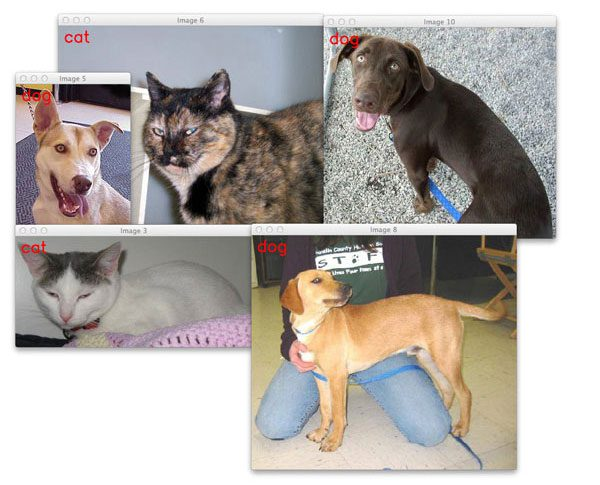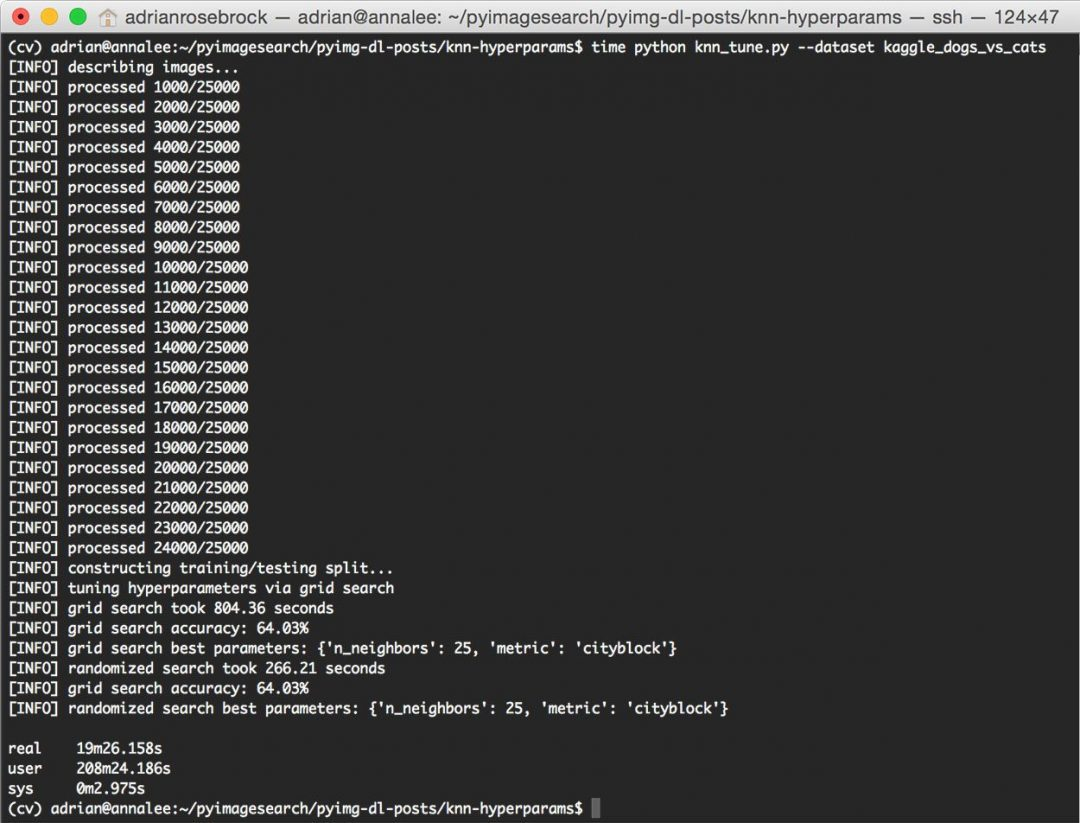# How to tune hyperparameters with Python and scikit-learnIn last week’s post, I introduced the k-NN machine learning algorithm which we then applied to the task of image classification.

Using the k-NN algorithm, we obtained 57.58% classification accuracy on the Kaggle Dogs vs. Cats dataset challenge:Figure 1: Classifying an image as whether it contains a dog or a cat.

The question is: “Can we do better?”

Of course we can! Obtaining higher accuracy for nearly any machine learning algorithm boils down to tweaking various knobs and levels.

In the case of k-NN, we can tune k, the number of nearest neighbors. We can also tune our distance metric/similarity function as well.

Of course, hyperparameter tuning has implications outside of the k-NN algorithm as well. In the context of Deep Learning and Convolutional Neural Networks, we can easily have hundreds of various hyperparameters to tune and play with (although in practice we try to limit the number of variables to tune to a small handful), each affecting our overall classification to some (potentially unknown) degree.

Because of this, it’s important to understand the concept of hyperparameter tuning and how your choice in hyperparameters can dramatically impact your classification accuracy.

Looking for the source code to this post?

## How to tune hyperparameters with Python and scikit-learn

In the remainder of today’s tutorial, I’ll be demonstrating how to tune k-NN hyperparameters for the Dogs vs. Cats dataset. We’ll start with a discussion on what hyperparameters are, followed by viewing a concrete example on tuning k-NN hyperparameters.

We’ll then explore how to tune k-NN hyperparameters using two search methods: Grid Search and Randomized Search.

As our results will demonstrate, we can improve our classification accuracy from 57.58% to over 64%!

### What are hyperparameters?

Hyperparameters are simply the knobs and levels you pull and turn when building a machine learning classifier. The process of tuning hyperparameters is more formally called hyperparameter optimization.

So what’s the difference between a normal “model parameter” and a “hyperparameter”?

Well, a standard “model parameter” is normally an internal variable that is optimized in some fashion. In the context of Linear Regression, Logistic Regression, and Support Vector Machines, we would think of parameters as the weight vector coefficients found by the learning algorithm.

On the other hand, “hyperparameters” are normally set by a human designer or tuned via algorithmic approaches. Examples of hyperparameters include the number of neighbors k in the k-Nearest Neighbor algorithm, the learning rate alpha of a Neural Network, or the number of filters learned in a given convolutional layer in a CNN.

In general, model parameters are optimized according to some loss function, while hyperparameters are instead searched for by exploring various settings to see which values provided the highest level of accuracy.

Because of this, it tends to be easier to tune model parameters (since we’re optimizing some objective function based on our training data) whereas hyperparameters can require a nearly blind search to find optimal ones.

### k-NN hyperparameters

As a concrete example of tuning hyperparameters, let’s consider the k-Nearest Neighbor classification algorithm. For your standard k-NN implementation, there are two primary hyperparameters that you’ll want to tune:

1. The number of neighbors k.
2. The distance metric/similarity function.

Both of these values can dramatically affect the accuracy of your k-NN classifier. To demonstrate this in the context of image classification, let’s apply hyperparameter tuning to our Kaggle Dogs vs. Cats dataset from last week.

Open up a new file, name it knn_tune.py , and insert the following code:

Lines 2-12 start by importing our required Python packages. We’ll be making heavy use of the scikit-learn library, so if you do not have it installed, make sure you follow these instructions.

We’ll also be using my personal imutils library, so make sure you have it installed as well:

Next, we’ll define our extract_color_histogram  function:

This function accepts an input image  along with a number of bins  for each channel of the image.

We convert the image to the HSV color space and compute a 3D color histogram to characterize the color distribution of the image (Lines 17-19).

This histogram is then flattened into a single 8 x 8 x 8 = 512-d feature vector that is returned to the calling function.

For a more detailed review of this method, please refer to last week’s blog post.

Lines 34-39 handle parsing our command line arguments. We only need two switches here:

• --dataset : The path to our input Dogs vs. Cats dataset from the Kaggle challenge.
• --jobs : The number of processors/cores to utilize when computing the nearest neighbors for a particular data point. Setting this value to -1  indicates all available processors/cores should be used. Again, for a more detailed review of these arguments, please refer to last week’s tutorial.

Line 43 grabs the paths to our 25,000 input images while Lines 46 and 47 initializes the data  list (where we’ll store the color histogram extracted from each image) and labels  list (either “dog” or “cat” for each input image), respectively.

Next, we can loop over our imagePaths  and describe them:

Line 50 starts looping over each of the imagePaths . For each imagePath , we load it from disk and extract the label  (Lines 53 and 54).

Now that we have our image , we compute a color histogram (Line 58), followed by updating the data  and labels  lists (Lines 59 and 60).

Finally, Lines 63 and 64 display the feature extraction progress to our screen.

In order to train and evaluate our k-NN classifier, we’ll need to partition our data  into two splits: a training split and a testing split:

Here we’ll be using 75% of our data for training and the remaining 25% for evaluation.

Finally, let’s define the set of hyperparameters we are going to optimize over:

The above code block defines a params  dictionary which contains two keys:

• n_neighbors : The number of nearest neighbors k in the k-NN algorithm. Here we’ll search over the odd integers in the range [0, 29] (keep in mind that the np.arange  function is exclusive).
• metric : This is the distance function/similarity metric for k-NN. Normally this defaults to the Euclidean distance, but we could also use any function that returns a single floating point value representing how “similar” two images are. In this case, we’ll search over both the Euclidean distance and Manhattan/City block distance.

Now that we have defined the hyperparameters we want to search over, we need a method that actually applies the search. Luckily, the scikit-learn library already has two methods that can perform hyperparameter search for us: Grid Search and Randomized Search.

As we’ll find out, it’s normally preferable to used Randomized Search over Grid Search in nearly all circumstances.

### Grid Search hyperparameters

The Grid Search tuning algorithm will methodically (and exhaustively) train and evaluate a machine learning classifier for each and every combination of hyperparameter values.

In this case, given 16 unique values of k and 2 unique values for our distance metric, a Grid Search will apply 30 different experiments to determine the optimal value.

You can see how a Grid Search is performed in the following code segment:

The primary benefit of the Grid Search algorithm is also it’s major drawback: as an exhaustive search your number of possible parameter values explodes as both the number of hyperparameters and hyperparameter values increases.

Sure, you get to evaluate each and every combination of hyperparameter — but you pay a cost — it’s a very time consuming cost. And in most cases, it’s hardly worth it.

As I explain in the “Use Randomized Search for hyperparameter tuning (in most situations)” section below, there are rarely just one set of hyperparameters that obtain the highest accuracy.

Instead, there are “hot zones” of hyperparameters that all obtain near identical accuracy. The goal is to explore as many of these “zones” of hyperparameters a quickly as possible and locate one of these “hot zones”. It turns out that a random search is a great way to do this.

### Randomized Search hyperparameters

The Random Search approach to hyperparameter tuning will sample hyperparameters from our params  dictionary via a random, uniform distribution. Given a set of randomly sampled parameters, a model is then trained and evaluated.

We perform this set of random hyperparameter sampling and model construction/evaluation for a preset number of times. You set the number of evaluations to be as long as you’re willing to wait. If you’re impatient and in a hurry, make this value low. And if you have the time to spend on a longer experiment, increase the number of iterations.

In either case, the goal of a Randomized Search is to explore a large set of possible hyperparameter spaces quickly — and the best way to accomplish this is via simple random sampling. And in practice, it works quite well!

You can find the code to perform a Randomized Search of hyperparameters for the k-NN algorithm below:

### Hyperparameter tuning with Python and scikit-learn results

To tune the hyperparameters of our k-NN algorithm, make sure you:

From there, you can execute the following command to tune the hyperparameters:

You’ll probably want to go for a nice walk and stretch your legs will the knn_tune.py  script executes.

On my machine, it took 19m 26s to complete, with over 86% of this time spent Grid Searching:Figure 2: Applying a Grid Search and Randomized to tune machine learning hyperparameters using Python and scikit-learn.

As you can see from the output screenshot, the Grid Search method found that k=25 and metric=’cityblock’ obtained the highest accuracy of 64.03%. However, this Grid Search took 13 minutes.

On the other hand, the Randomized Search obtained an identical accuracy of 64.03% — and it completed in under 5 minutes.

Both of these hyperparameter tuning methods improved our classification accuracy (64.03% accuracy, up from 57.58% from last week’s post) — but the Randomized Search was much more efficient.

### Use Randomized Search for hyperparameter tuning (in most situations)

Unless your search space is small and can easily be enumerated, a Randomized Search will tend to be more efficient and yield better results faster.

As our experiments demonstrated, Randomized Search was able to obtain 64.03% accuracy in < 5 minutes while an exhaustive Grid Search took a much longer 13 minutes to obtain an identical 64.03% accuracy — that’s a 202% increase in evaluation time for identical accuracy!

In general, there isn’t just one set of hyperparameters that obtains optimal results — instead, there are usually a set of them that exist towards the bottom of a concave bowl (i.e., the optimization surface).

As long as you hit just one of these parameters towards the bottom of the bowl, you’ll still obtain the same accuracy as if you enumerated all possibilities along the bowl. Furthermore, you’ll be able to explore various regions of this bowl faster by applying a Randomized Search.

Overall, this will lead to faster, more efficient hyperparameter tunings in most situations.

## Summary

In today’s blog post, I demonstrated how to tune hyperparameters to machine learning algorithms using the Python programming language and the scikit-learn library.

First, I defined, the difference between standard “model parameters” and the “hyperparameters” that need to be tuned.

From there, we applied two methods to tune hyperparameters:

1. An exhaustive Grid Search
2. A Randomized Search

Both of these hyperparameter tuning routines were then applied to the k-NN algorithm and the Kaggle Dogs vs. Cats dataset.

Each respective tuning algorithm obtained identical accuracy — but the Randomized Search was able to obtain this increase of accuracy in a fraction of the time!

In general, I highly encourage you to use Randomized Search when tuning hyperparameters. You’ll often find that there is rarely just one set of hyperparameters that obtains optimal accuracy. Instead, there are “hot zones” of hyperparameters that will obtain near-identical accuracy — the goal is to explore as many zones and try to land on one of these zones as fast as possible.

Given no a priori knowledge of good hyperparameter choices, a Randomized Search to hyperparameter tuning is the most optimal way to find reasonable hyperparameter values in a short amount of time as it allows you to explore many areas of the optimization surface.

Anyway, I hope you enjoyed this blog post! I’ll be back next week to discuss the basics of linear classification (and the role it plays in Neural Networks and image classification).

But before you go, be sure to signup for the PyImageSearch Newsletter using the form below to be notified when future blog posts are published!If you would like to download the code and images used in this post, please enter your email address in the form below. Not only will you get a .zip of the code, I’ll also send you a FREE 17-page Resource Guide on Computer Vision, OpenCV, and Deep Learning. Inside you'll find my hand-picked tutorials, books, courses, and libraries to help you master CV and DL! Sound good? If so, enter your email address and I’ll send you the code immediately!

### 7 Responses to How to tune hyperparameters with Python and scikit-learn

1.Paulo August 16, 2016 at 5:50 am #

Of course we can!

2.John November 29, 2016 at 4:51 pm #

Great Post! Thanks for the great content.

•Adrian Rosebrock December 1, 2016 at 7:42 am #

Thanks John, I’m happy you found it useful!

3.Sah San August 20, 2017 at 9:54 am #

Hi,
How do we know which hyperparameter to include in either the gridsearchcv or randomsearch?
For example, decision trees has many hyperparameter such as
min_samples_split, min_samples_leaf, min_weight_fraction_leaf, max_features,  max_leaf_nodes.

I don’t know if we can include all these hyperparameter .
Any comment would be appreciated. Thanks

•Adrian Rosebrock August 21, 2017 at 3:40 pm #

Typically you would research the model you want to apply and the associated hyperparameters. This normally involves reading the original publication(s) where the authors share which hyperparameters are the most important.

•Sah San August 23, 2017 at 6:06 pm #

Thank you. It was so helpful

4.nawal November 15, 2018 at 8:49 am #

I thinks that’s in line 101you want to type:

print(“[INFO] randomized search accuracy: {:.2f}%”.format(acc * 100))

Not
print(“[INFO] grid search accuracy: {:.2f}%”.format(acc * 100))

??